# Quiz 11: Criticisms of Absorption Cost Systems: Inaccurate Product Costs

Income Statement: It is a part of the books of a company and is a most important element as it shows the performance of a company. It shows the net profit by subtracting all the expenses from the income that incurred during the fiscal year. Overhead Cost: It can be referred to as an accounting term which states all the indirect expenses incurred by an organization for operating a business. These expenses are not directly related to the manufacturing of a product or rendering of a service.a. Prepare the income statement for two ball types, using MS-excel as shown below: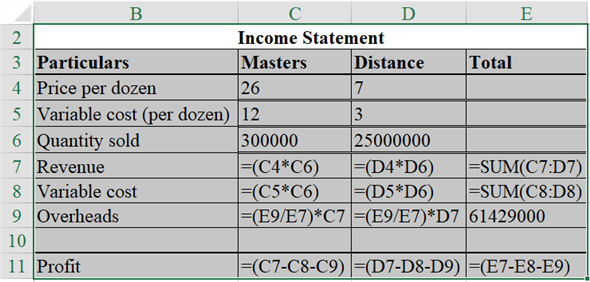The result of the above excel table is as follows: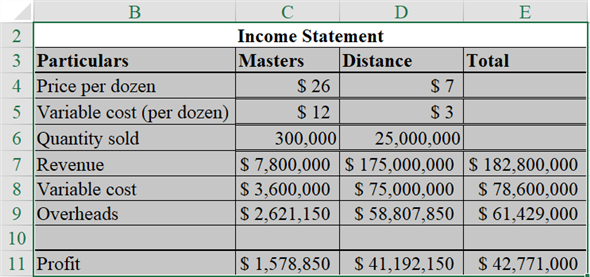Hence, the net income of Masters is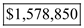and the net income of Distance is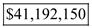. b. Prepare the income statement for two ball types, using MS-excel as shown below: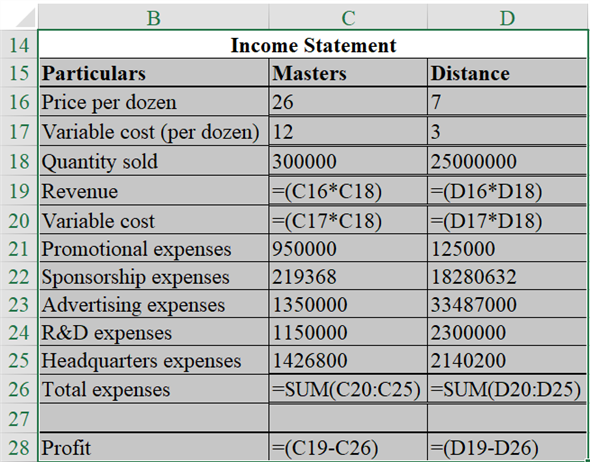The result of the above excel table is as follows: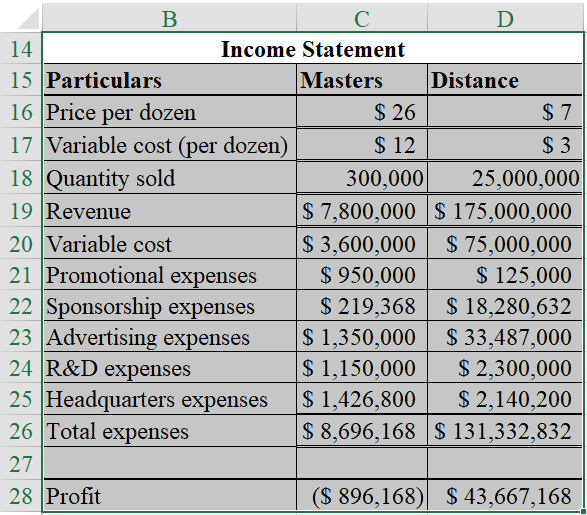Hence, the net income of Masters is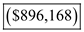and the net income of Distance is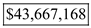Working notes: 1. Prepare the schedule showing the allocation of sponsorship expenses, using MS-excel as shown below: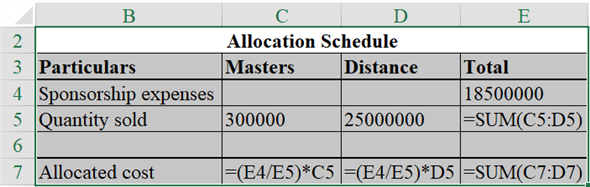The result of the above-excel table is as follows: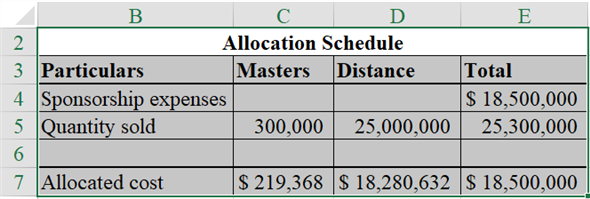Hence, the sponsorship expenses allocated to masters and Distance is \$219,368 and \$18,280,632 respectively. 2. Compute the R D expenses allocated to master, using the equation as shown below:Hence, the R D expenses allocated to master is \$1,150,000. 3. Compute the R D expenses allocated to distance, using the equation as shown below:Hence, the R D expenses allocated to distance is \$2,300,000. 4. Compute the ball division headquarters expenses allocated to master, using the equation as shown below:Hence, the headquarters expenses allocated to master is \$1,426,800. 5. Compute the ball division headquarters expenses allocated to distance, using the equation as shown below:Hence, the headquarters expenses allocated to distance is \$2,140,200. c. Activity-based costing (ABC) is a method of distribution of overheads between various jobs or products. Under this system, the main activities of the business are determined and divided into cost pools. Cost drivers of these main activities have been decided and estimated. Then, the cost pools of these activities are divided with the significant cost drivers. The profitability of the two ball type has changed when the company uses the ABC system instead of plant-wide rate. The profitability of master type ball is decreased and the profitability of distance type ball is increased.
Income Statement: It is a part of the books of a company and is a most important element as it shows the performance of a company. It shows the net profit by subtracting all the expenses from the income that incurred during the fiscal year Absorption Costing: It is a method used in the cost accounting system, under which all the expenses which are related to the production or manufacturing of a product are entered or taken into consideration. Under this method, all the expenses relating to the manufacturing are absorbed by the units that are being produced by the company at that point in time.a.In the present case, the information regarding the operations which were undertaken in the last year by the company in summarized form are provided. Prepare the required Income Statement of the company with the help of MS-Excel as follows: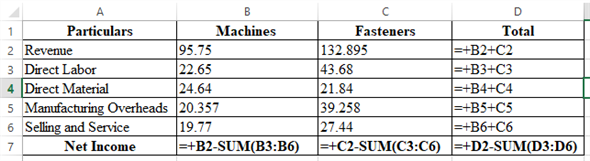The result of the above calculation is as follows: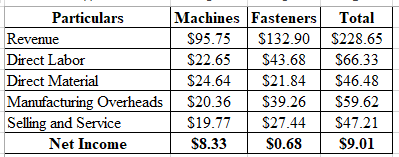Therefore the Net Income of the Machine and Fasteners comes out to be \$8.33 and \$0.68 respectively. b.First, prepare a table for the allocation and bifurcation of, manufacturing overheads as follows: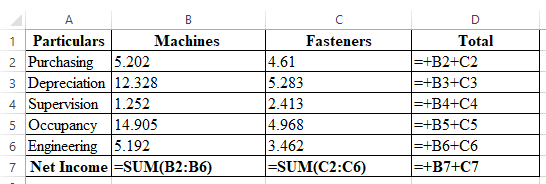The result of the above calculation is as follows: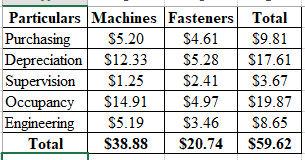Now, finally compute the revised net income of the company as follows: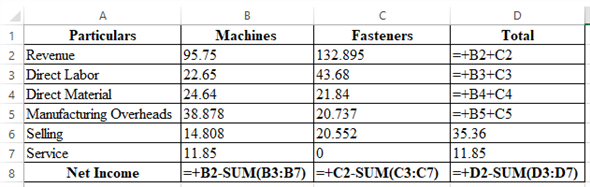The result of the above calculation is as follows: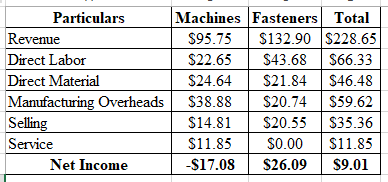Therefore the Net Income of the company comes out to be -\$17.08 for Machines and \$26.09 for fasteners. c.The major difference in the profits of the company under both the methods is due to the attachment of depreciation or the whole machinery in such calculations. Such attachment of machinery in the calculations resulted in profits for the company in the first alternative. But the same has resulted in negative net income for the company by charging the amount of depreciation in it. d.In the present case, the second alternative as adopted by the company for the computation of the net income is better. This is because it has accurately charged the indirect costs of the company in a very unique manner. Thus the computation of net income is more accurate even if the products under which the company is dealing are interdependent on each other. In the latter alternative, the indirect costs were charged appropriately and hence forcing the Company to charge higher prices for the same. But under the changed method, the company has overcome the lacuna and has appropriately estimated the expenses and has also computed the amount of income accurately.